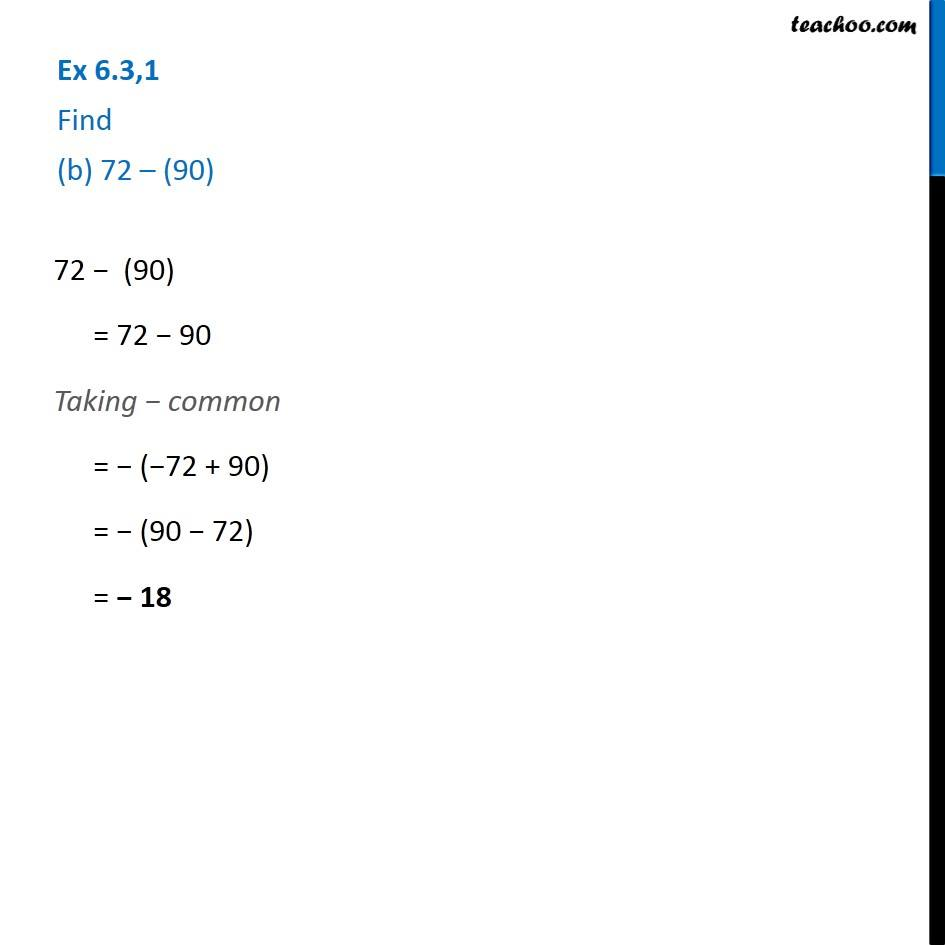1. Chapter 6 Class 6 Integers
2. Serial order wise
3. Ex 6.3

Transcript

Ex 6.3,1 Find (b) 72 – (90) 72 − (90) = 72 − 90 Taking − common = − (−72 + 90) = − (90 − 72) = − 18